# Taming POV-Ray - Part 0: Fundamentals

#### by G. Moran, 10 Feb 2022

So you want to get into 3D graphics, and maybe try using POV-Ray if you dare? Good. You came to the right place. I was in your shoes about a year ago, and it took me quite some time to find the information I needed and learn it, before I went on and created the art you saw on your way here... Now I'm gonna pass onto you the knowledge and experience I gained, hopefully in the simplest way possible, so you too can create 3D art regardless of your background. It's not as intimidating as you may think. There's a learning curve, sure, but once you get over the basics it becomes progressively easier. You'll see...

This article will cover the fundamentals of 2D, which you need to learn before jumping into the world of 3D.

## The Grid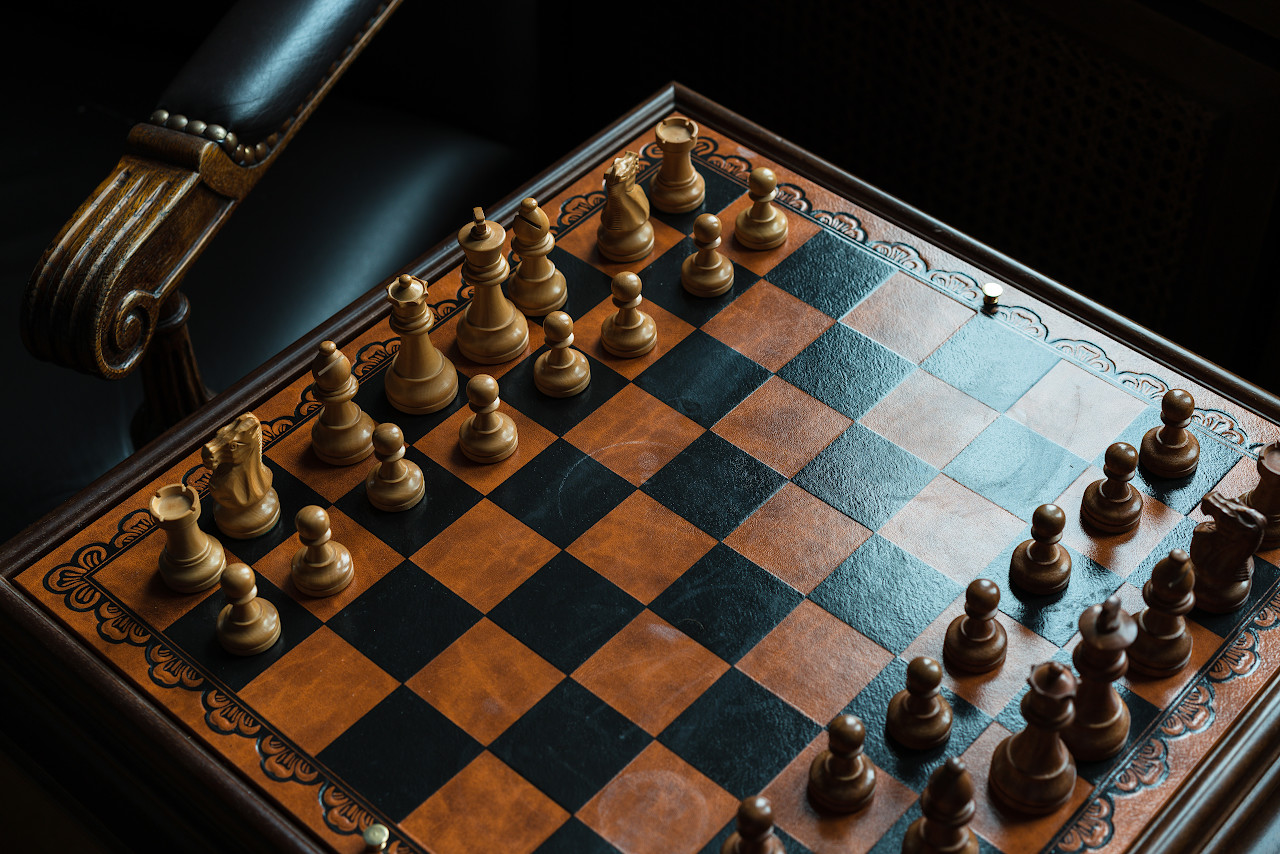##### Photo by Shannon Potter

Imagine standing on a checkered floor, like a room-sized chess board, and observing the black and white tiles under your feet. Every tile is a square with the same exact dimensions, right? Basically, you're standing on a "grid". A grid is made up of cells, like how the floor is made up of tiles, and we can consider each cell as a unit for measuring things like distance and size. For example:

• Alice is standing 2 units west and 3 units north of Bob
• The table is 4 units long and 2 units wide
• This room is 8 x 8 units squared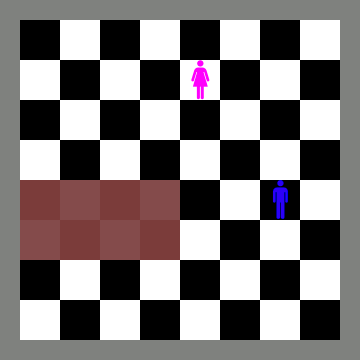Grid cells can also be used to find the position of things relative to a common point, we'll call that point the "origin". Going back to the checkered floor, let's imagine a point at the south west corner of the room, we'll use that point as the origin. Then, with a virtual compass in hand, we'll refer to the position of any object using two numbers called the "coordinates"; those denote how far east and how far north is the object from the origin. For example:

• Alice is standing at `(4.5, 6.5)` which means `4.5` units east and `6.5` units north of the origin. Similarily, Bob is standing at `(6.5, 3.5)`
• A table's corners are at `(0, 2)` , `(0, 4)` , `(4, 4)` , `(4, 2)`, which are the south west, north west, north east, and south east corners respectively
• The origin is at `(0, 0)`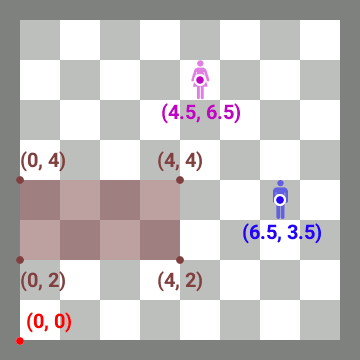## The Coordinate System

Now that we know what a 2D grid is, what an origin is, and how to write coordinates, let's standardize our system... Instead of using a virtual compass, we'll draw two imaginary "axes" or rulers that are perpendicular to each other, both extending out of the origin; one ruler called "the `x` axis" extending towards the east, and another ruler called "the `y` axis" extending towards the north. Now we can easily find out the coordinates of any object by simply observing its position relative to the 2 axes; we'll see how far along `x` (east) and how far along `y` (north) is the object located. From now on we'll be referring to coordinates as an `x` coordinate and `y` coordinate, instead of east coordinate and north coordinate.

This system with the 2 axes is called the "coordinate system". We will be adding a third axis to it later, but for now, let's draw a new grid and put Alice, Bob, and the table on it.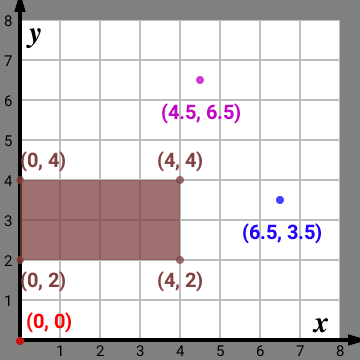The coordinate system doesn't only contain positive coordinates though, it can also contain negative coordinates. Let's extend the 2 axes in the "opposite" directions, so that we can also go west and south from the origin. Points that are west of the origin have a negative `x` coordinate, and points that are south of the origin have a negative `y` coordinate. For example:

• Charlie is standing at `(-3.5, 4.5)` and Dave at `(4.5, -2.5)`
• A rug's corners are at `(-7, 2)` , `(-4, 2)` , `(-4, -2)` , `(-7, -2)`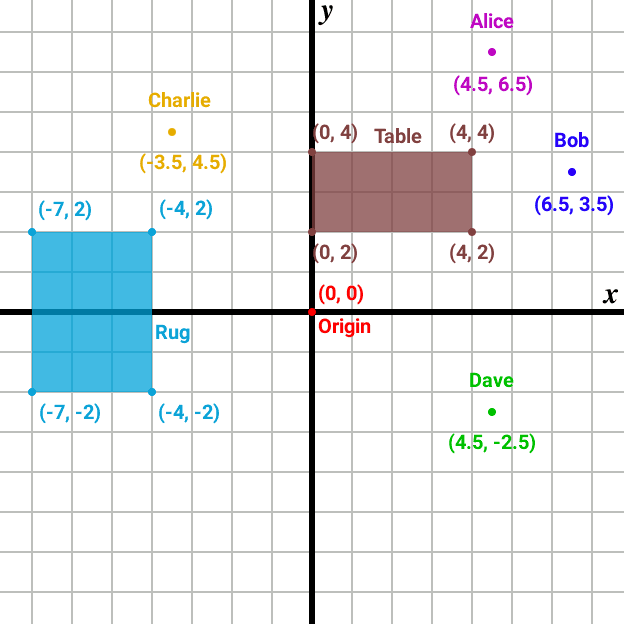## Basic 2D Shapes

In the examples above we have seen two types of 2D shapes; points and rectangles. Let's go over each of those as well as other basic shapes.

### Point

The point is the most basic shape we have, except it's not a "shape" in the literal sense because it doesn't have a width or height, and doesn't occupy any real space. Points are represented by dots, simply to mark a certain location on a grid.

We denote a point by a single pair of coordinates, for example `(4, 2)`.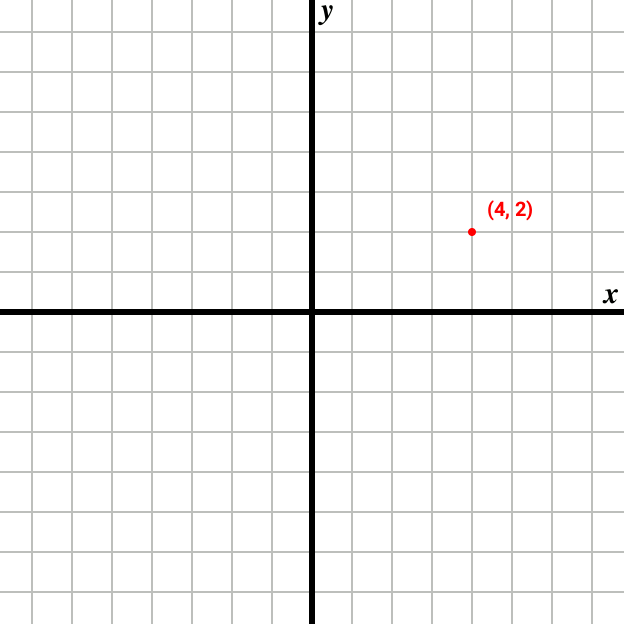### Line

A line is a straight connector between 2 points. It doesn't have a width or height or "thickness", and doesn't occupy any real space.

We denote a line by 2 pairs of coordinates, corresponding to the 2 points that connect to form it, for example `(4, 2)` , `(0, 4)`.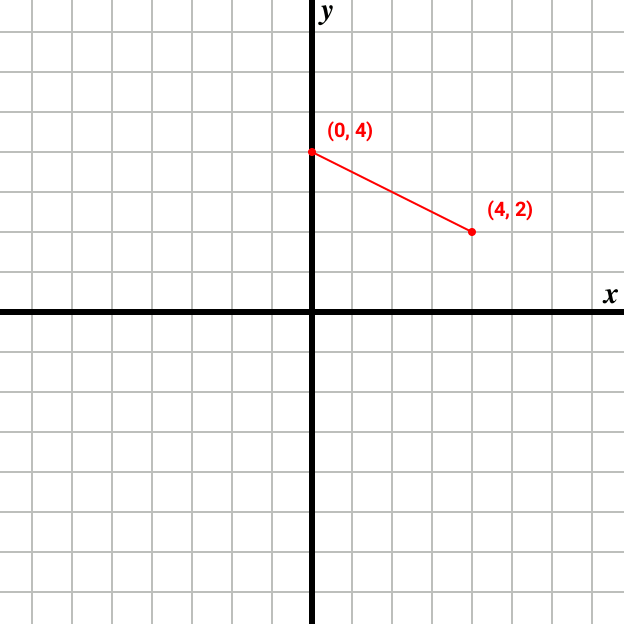### Triangle

The triangle is the first basic shape we have that occupies real measurable space (i.e. area), it's made up of 3 points connected to each other by straight lines, and every point is directly connected to the other 2. The triangle is a "closed" shape, because it's completely surrounded by lines without gaps, we call those lines "edges" because they are drawn exactly where the shape ends.

We denote a triangle by 3 pairs of coordinates, corresponding to its 3 points, for example `(4, 2)` , `(0, 4)` , `(3, 5)`.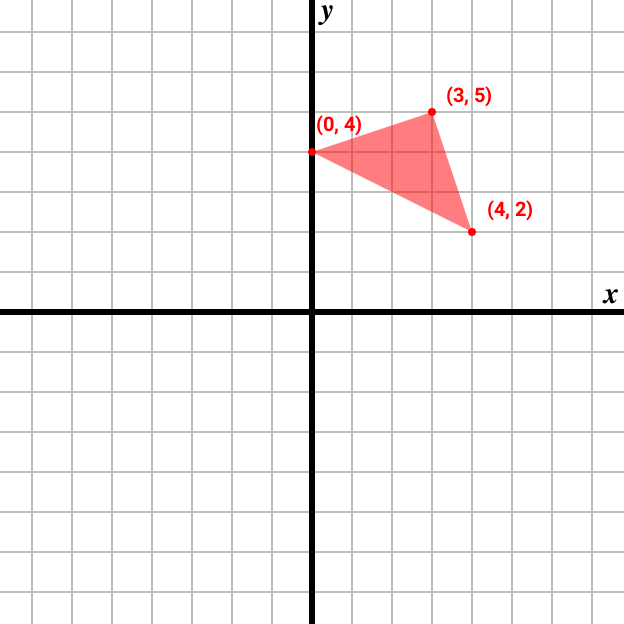### Rectangle

A rectangle is another closed shape made up of 4 different points connected to each other by straight lines, however every point is directly connected only to 2 other points. The 4 edges that make up the rectangle are divided into 2 horizontal edges and 2 vertical edges.

We denote a rectangle by 4 pairs of coordinates, corresponding to its 4 points in an order that creates a closed shape, for example `(0, 2)` , `(0, 4)` , `(4, 4)` , `(4, 2)`, which gives us our south west, north west, north east and south east points respectively.

An alternative notation for a rectangle is only 2 pairs of coordinates, such that these coordinates correspond to 2 points that are opposite to each other, for example `(0, 4)` , `(4, 2)`, which gives us our north west and south east points respectively. Notice how the `x` coordinate is different across the two pairs, and so is the `y` coordinate? We will be using this alternative notation from now on.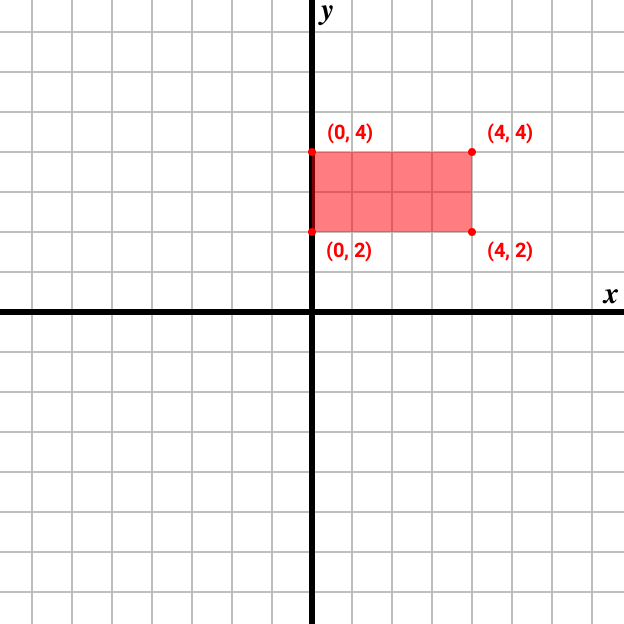### Circle

Circles are a little tricky, because they are represented only by a single point and a number called the "radius". Circles are closed but have no edges.

We start with a point that's gonna be the center of the circle, then we choose a number that's pretty much how "big" our circle is gonna be, we'll call that number the radius. Then, with a virtual pen, we draw a circular outline around the center point, such that the pen remains away from the center point by the length of the radius... This roughly means that if we measure the distance between the center point and any other point on the outline of the circle, the distance will always be equal to the radius.

We denote a circle by a pair of coordinates for the center point and a single radius value, for example `(4, 5) , 2`.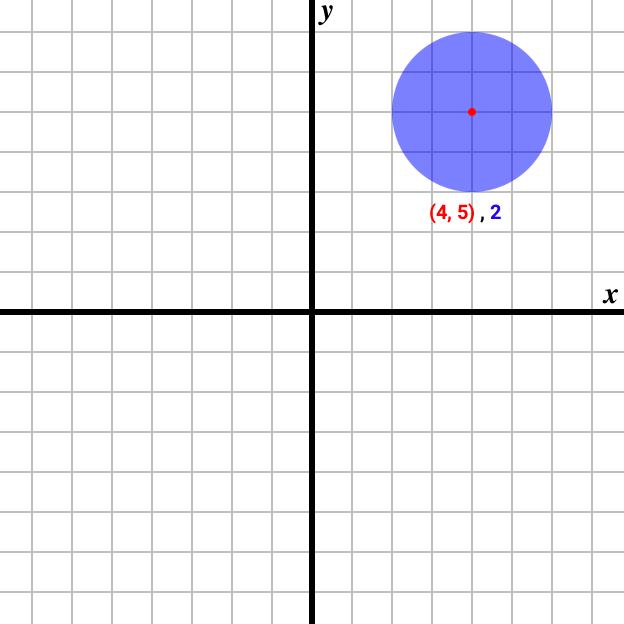### Bézier Curve

A curve in general is a non-straight line, a "bent" line, if you will. Like the straight line it has no width, height, or thickness. There are many different ways to represent a curve, each with their own mathematical formula(e), but we'll only briefly cover one; the cubic Bézier curve.

We're gonna skip the math here, all you need to know is that any cubic Bézier curve is represented by 4 points; the 1st and 4th points are where the curve ends, and the 2nd and 3rd points control the shape of the curve. A cubic Bézier curve will always pass through the 1st and 4th points, but only rarely pass through the other 2 points. You can experiment with drawing cubic Bézier curves using the path tool in GIMP, or the equivalent tool in other image manipulation programs.

We denote a cubic Bézier curve by 4 pairs of coordinates in the order specified above, for example `(1, 2)` , `(2, 6)` , `(4, 1)` , `(6, 4)`. That's 1 end point, 2 control points, then 1 more end point.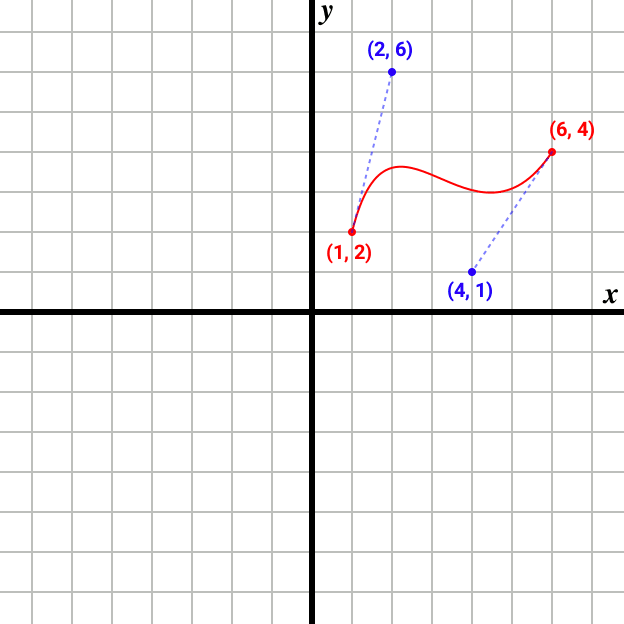## 2D Transformations

Time to mess with shapes! This part is more challenging than all the above, so take your time to fully understand it.

### Translation

Translating basically means moving or displacing, by specifying how many units along `x` and along `y` we want to move. We denote translation using a regular pair of coordinates, for example:

• Translating Alice by `(2, -3)` will place her at the same position as Bob
• Translating Charlie by `(8, -7)` will place her at the same position as Dave
• Translating Dave by `(-10, 2)` will place him on the rug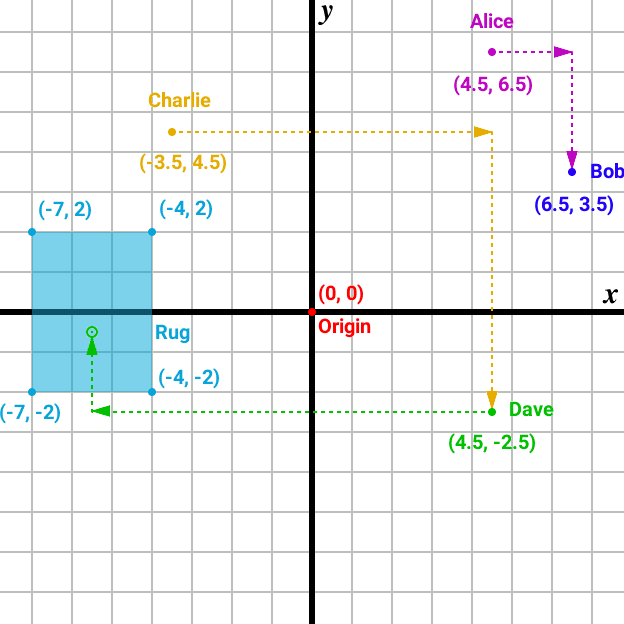### Rotation

Rotation is measured in something called "degrees", and to explain what those are we have to look at clocks. Imagine a regular "non-digital" clock with 2 hands, both pointing at 12. Let's focus on the minute hand... After a quarter of an hour, the minute hand will have rotated and will be pointing at 3, another quarter of an hour and the hand will be pointing at 6, then at 9, then back at 12 again after a full hour had passed.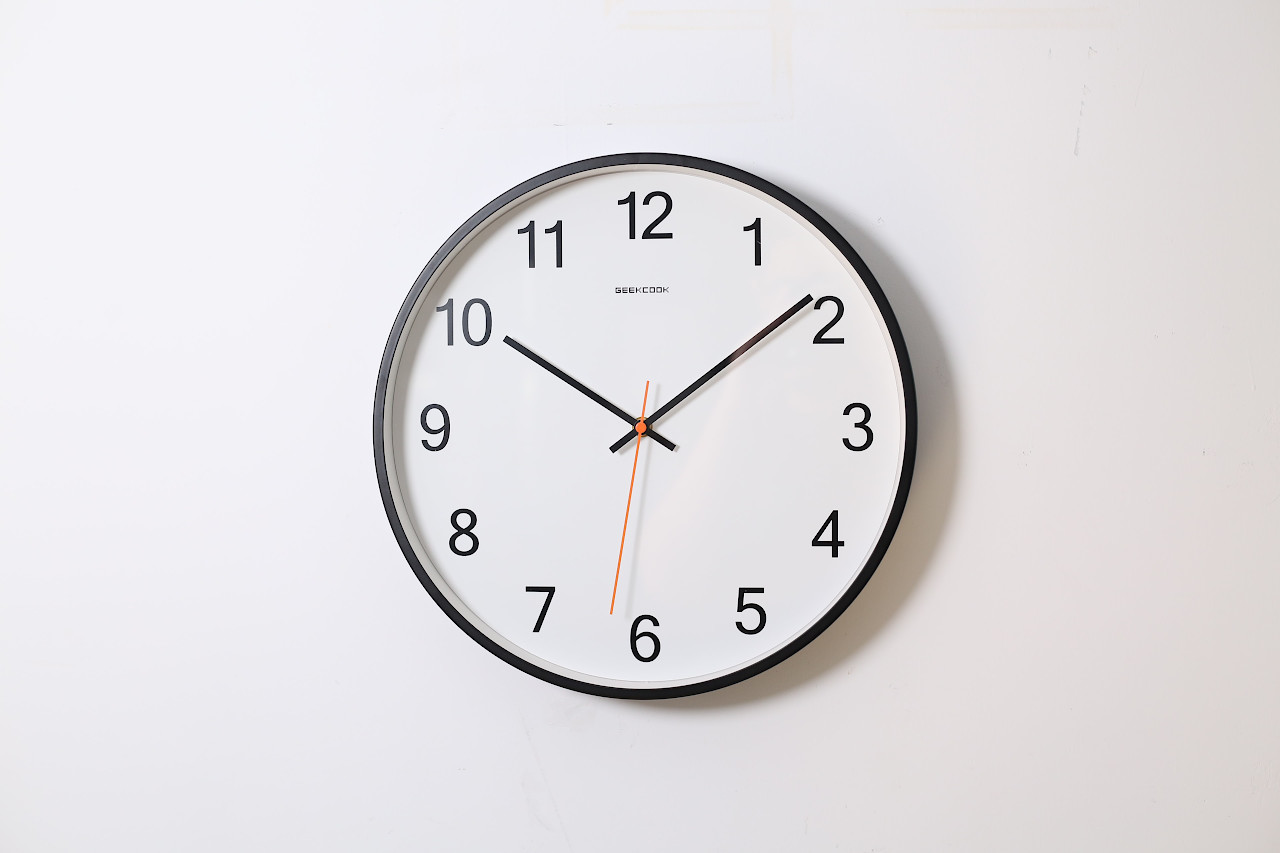##### Photo by Ocean Ng

So what happened exactly? The minute hand rotates around the center of the clock, and after the passing of every hour the hand makes a "full" rotation and goes back to where it started, and the process repeats. Let's quantify all this rotation; whenever a hand makes a "full" rotation it takes 360 "steps", if it makes half of a full rotation it takes 180 "steps" (360/2), and if it makes quarter of a full rotation it takes 90 "steps" (360/4) and so on. You see where I'm going with this?

They're not "steps" though, they're "degrees", and a group of degrees is called an "angle". Whereas a clock has 12 hours marked on it, a circle has 360 degrees. So the minute hand rotates by an angle of 90 degrees to go from 12 to 3, then rotates by another angle of 90 degrees to go from 3 to 6, having now rotated a total of 180 degrees, then by rotating another angle of 180 degrees it reaches 12 again and completes a 360 degree rotation...

But wait, if a full rotation is 360 degrees, does that mean that the next full rotation is 720 degrees, and the next 1080 and so on? Technically yes, but we don't stack up angles, we reset the counter to 0 after completing 360 degrees. So we count 1, 2, 3, ..., 357, 358, 359, 0, 1, 2, 3...etc.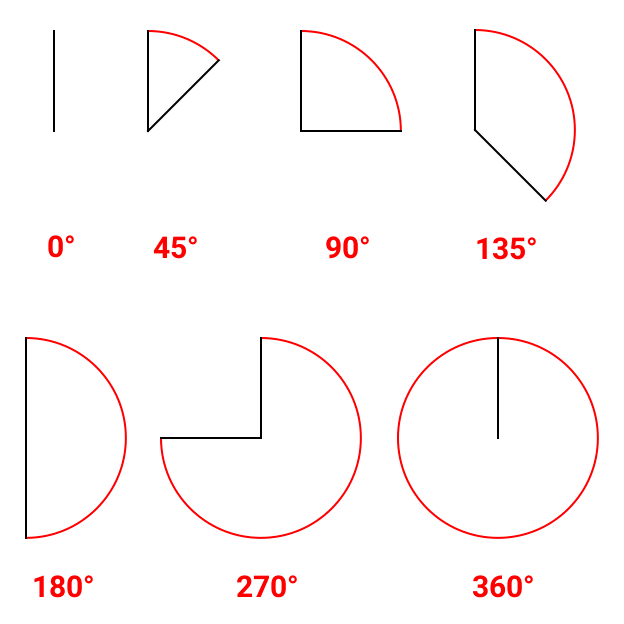So far all our rotation has been clockwise (i.e. in the same direction the clock hands rotate), but we can also rotate in the "opposite" direction; anti-clockwise. When we do this the angle keeps decreasing, and when it decreases below zero it becomes negative... Because of the circular nature of angles, every negative angle has an equivalent positive angle, and vice-versa. For example +90 is the same as -270, similarily +225 is the same as -135, and -180 is the same as +180.

Notice something here? If you add up the value of any 2 equivalent angles, ignoring the negative sign, you get 360. For example `90 + 270 = 360`, and `225 + 135 = 360`... That way it becomes trivial to find the negative equivalent of any positive angle, and vice-versa.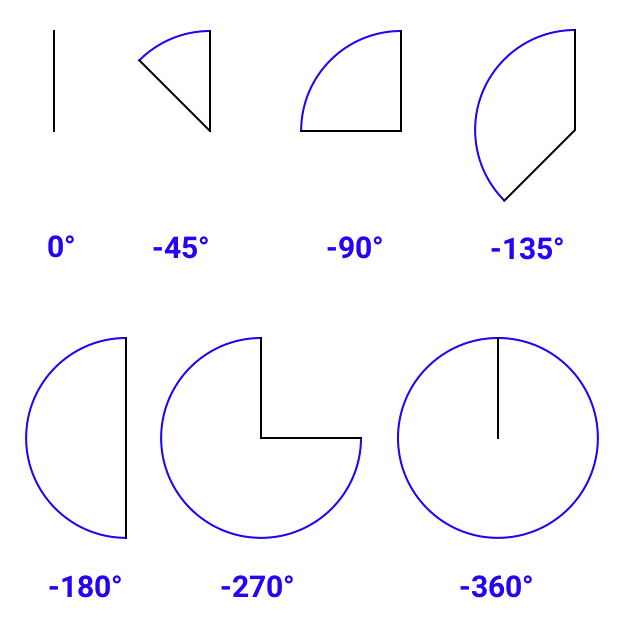Going back to the clock and the minute hand pointing at 12, if we stick a pin close to the "near" end of the hand (i.e. the fixed end), and another pin near the "far" end of the hand (i.e. the end that points at numbers), where do you think their position will be after 20 minutes had passed?

Surely, the "near" pin will have barely moved, and the "far" pin will have moved an awful lot. That's because objects travel a longer distance the farther they are from the center of rotation, which in our case is the center of the clock.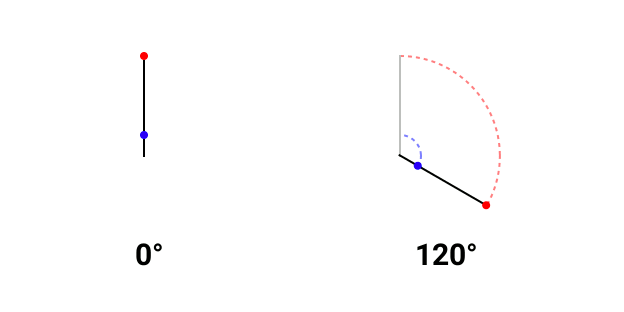Now it's time to standardize our system yet again. Following the coordinate system, the center of rotation is always the origin, just like the center of the clock... Any rotation will cause objects placed near the origin to travel shorter distances, and objects placed far from the origin to travel longer distances.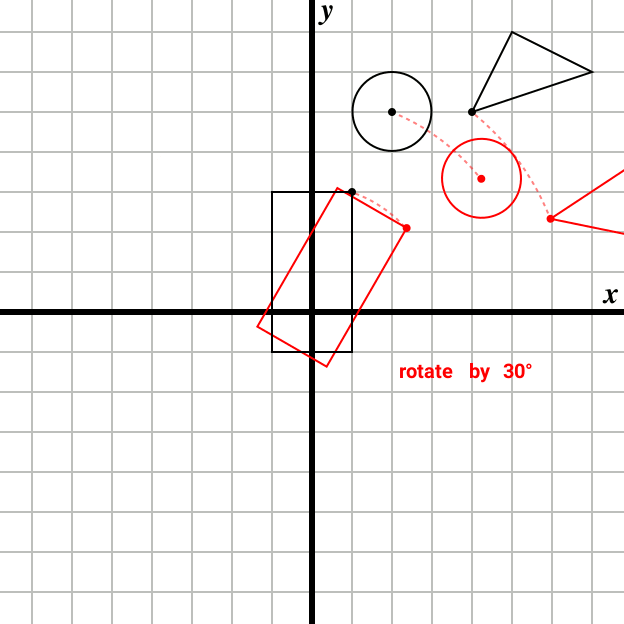So what if we want to rotate an object around a different point than the origin? In that case we bring that point to the origin by translating the object accordingly, then we do the rotation, then we "translate back" by undoing the first translation.

For example if there's an object at `(3, 4)`, and we want to rotate it by 45 degrees around the point `(3, 4)` instead of the origin, we have to first translate the object by `(-3, -4)`, rotate the object by 45 degrees, then translate the object by `(3, 4)`.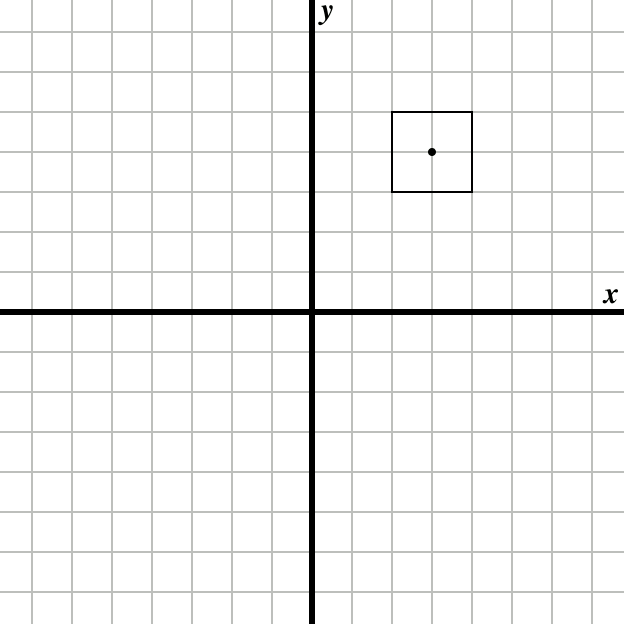### Scaling

Scaling is basically enlarging or shrinking an object, by some amount called the "factor". A scaling factor is how much we're enlarging or shrinking an object; if the factor is more than 1 the object will be enlarged, if the factor is less than 1 but more than 0 the object will be shrunk, and if the factor is 1 then the object's size won't change. For example scaling an object by a factor of 2 will double its size, and scaling the object by 0.5 will halve its size. Simple stuff, right?

Note that you cannot scale by a factor of 0.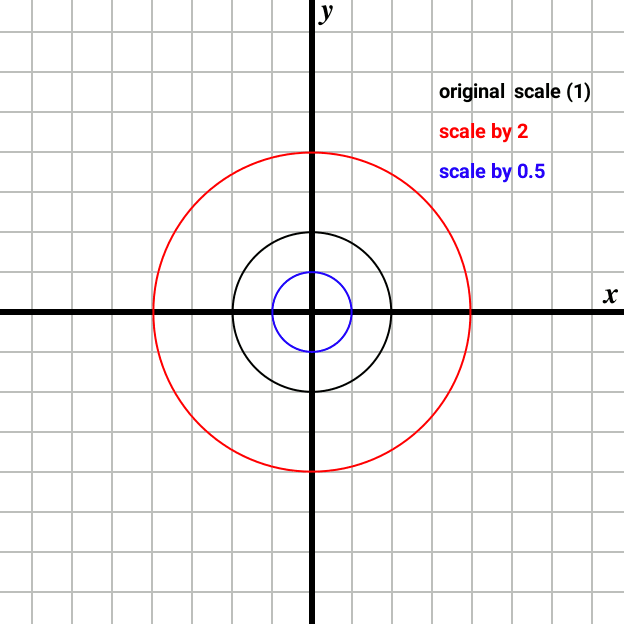We can scale an object along the `x` axis, the `y` axis, or both. Scaling by different factors in `x` and `y` will change the object's size but cause the object to be stretched, this is called "non-uniform" scaling. Scaling by the same factor in both `x` and `y` will change the object's size without stretching, this is called "uniform" scaling.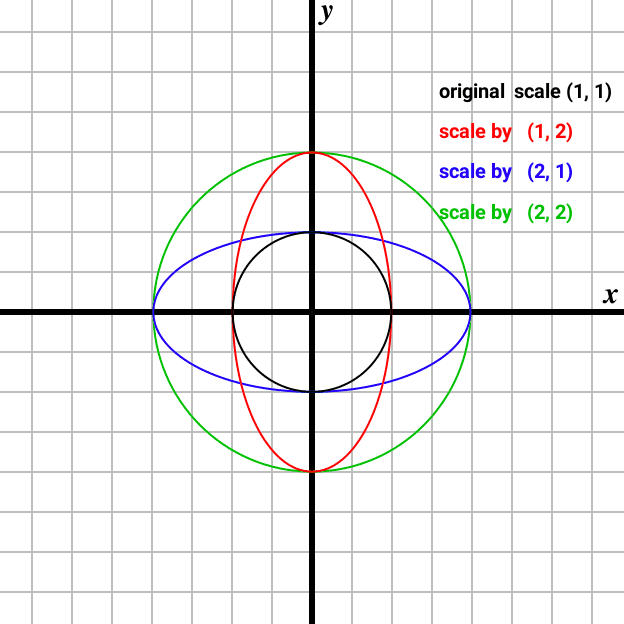Note that scaling, like rotation, always happens around the origin. So if you center an object around the origin and scale it by 2, it will double in size and remain centered around the origin. But if you place an object away from the origin and scale it by 2, it will double in size and the distance between it and the origin will also double.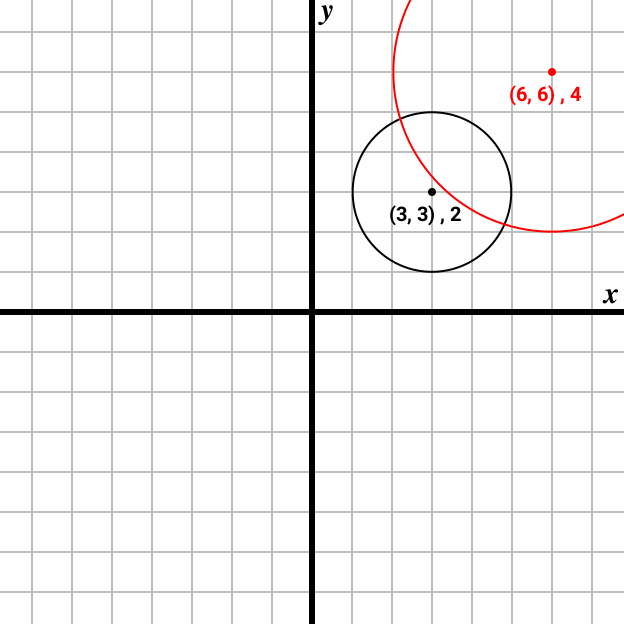You don't want to chase your objects around every time you scale them, so just translate them to the origin, scale them, then translate back, just like we did earlier with rotation.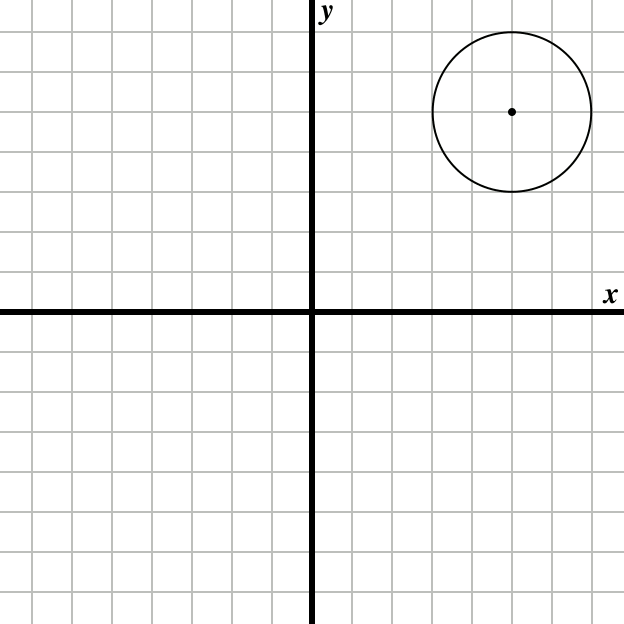Fun fact: you can scale by a negative factor! The result is the exact same as with a positive factor but the object is flipped or "inverted". If you scale by a negative factor in `x` the object will be mirrored, and if you scale by a negative factor in `y` the object will be upside down. If you scale by a negative factor in both `x` and `y` the object will be mirrored and upside down.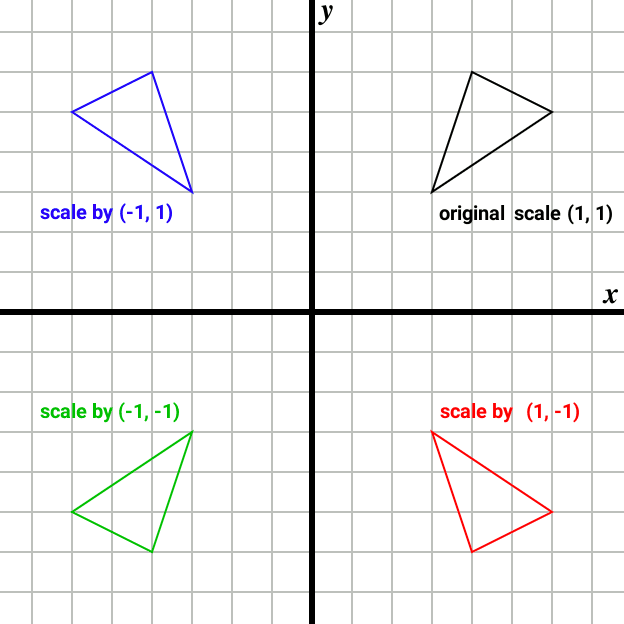## Wrapping Up

You should now know what is the coordinate system, how to place 2D shapes on it, and how to transform said shapes. In the next article we'll build on everything we learned here by adding a third dimension to the coordinate system, and exploring it using POV-Ray. You might want to get a headstart and download POV-Ray 2.2 (Windows/Linux) which we will be using.